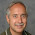Optimal portfolios and "diworsification"

Among the mathematical techniques that Operational Research scientists use is Quadratic Programming (QP).  It isn't often included in introductory courses, partly because it is hard to devise simple examples for classroom use.  I suspect that most courses including QP use portfolio optimisation as their introductory example.  This assumes that an investor has a finite sum to invest, and can divide it between a set of investments.  A great deal is known about these investments; the expected return on each, and the variance of each return, and the covariance of the returns between the investments.  Given these data, the expected return of the portfolio is a linear function of the decision variables (amount put into each investment).  The variance of that portfolio is a quadratic function of the same variables.  Then the simplest model is to minimise the variance subject to a constraint on the return.  Since this model was put forward in the research literature in the mid-20th century, it has been widely copied, criticised and developed.  The assumptions have been questioned, particularly the belief that one knows so much data about the investments, and the belief that the distribution of returns follows a normal distribution.

I read somewhere that the proponents of these models do not use their models in their investment holdings; does anyone know if this is so?

Recent studies by financial analysts have looked at theoretical investments to investigate the effect of the number of alternative investments on the variance of the return, and - as one might expect- the more possible investments, the smaller the variance.  For variance, read "risk".  But, and this seems intuitive, the variance tends to a limit, so that adding one new investment to a choice of five has much more effect than adding one new one to a choice of fifteen, and that in turn has more effect that adding one to a choice of twenty-five.  The phenomenon has been named "deworsification", a portmanteau word combining diversification and worse.

Analysts claim that once a stock portfolio reaches 20-30 holdings, there is little additional benefit to be gained from adding even more holdings.  Furthermore, a financial manager would like to have the chance to switch holdings, so as to grasp "investment opportunities", and the size of a holding in  a larger portfolio would not be enough to do this.  So there is another psychological advantage to this composition of holdings.

Discovery of the term "deworsification" made me wonder whether specialists in QP had been involved in the studies mentioned above.  One of my memories of QP models of portfolios is that the optimal solution often occurs at a flattish region of the objective function, so the optimal mix can be varied from the theoretical optimum without significant effect on the "risk", and this phenomenon ties in with the idea of "deworsification"; changing one decision variable from its optimal value to zero (taking it out of the model) has little effect on that measured "risk".

For many small investors, unit trusts are the way  to start.  Most unit trusts have more than 30 holdings, so they probably could have fewer holdings without serious effect on performance.  But, would a small investor be willing to put money in such a unit trust?  I wonder.

1.